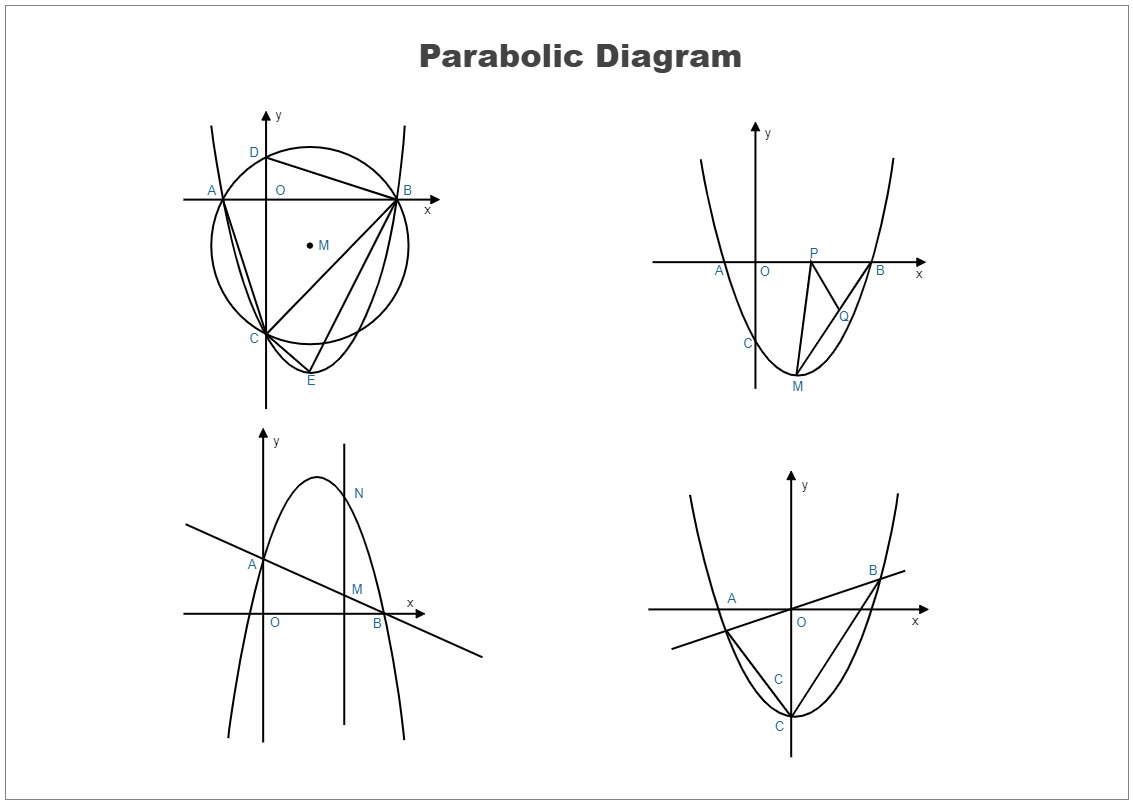Template Community / Parabolic Curve Diagram Example

# Parabolic Curve Diagram ExampleKiraaaa
Published on 2021-09-27
Edit OnlineHere is a parabolic curve example. According to mathematics, a plane curve with a U shape with a mirror-symmetry is a parabolic curve. A parabola has a point which is called a focus and a directrix. Any point present on the directrix line is equidistant from the focus of the parabola. In parabolic curve representation, consider the X-axis and Y-axis. The shape of curves on both sides of the Y-axis is the same. They are like mirror reflections. This property of the parabolic shape is known as symmetry. Learn more details from this diagram, or try to make yours with ease now!
Tag
science diagram
Mathematics Diagram
Report
0
144PostRecommended Templates